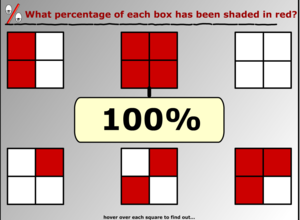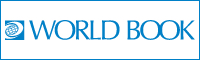School level

# Decimals and Percentages

## Decimals and Percentages by Scoilnet

Learn about decimals and percentages through interactive games and engaging activities.

Getting Started
Activities
Teachers

### Introduction to Percentages

http://www.mathsisfun.com/percentage.html Added: 11 Mar 2011 Contributor: Scoilnet Resource type: Game

This site introduces the user to the concept of percent. It takes them through a number of worked examples involving percentages and then lets them practice questions on their own.

JC Mathematics

#### How it maps to the curriculum

JC
Mathematics

Strand: 2. Numbers

Strand unit: 2. Equivalent Representation of Rational Num.

Suggestions for use: The teacher can use this site as a teaching aid when introducing percentages and then use the questions at the end to test student understanding of what they have been taught.

Asset type: Online Resource
Language: English
Special Needs:

### Introduction to Percentages

http://resources.hwb.wales.gov.uk/V...lly/percentages/eng/percentages.htm Added: 06 Nov 2007 Contributor: Scoilnet Resource type: Guide/notes / Presentation / Simulation

This is a great starting point to explain what percentages are and where they are used. .

5-6 Mathematics
JC Mathematics

#### How it maps to the curriculum

5-6
Mathematics

Strand: Number

Strand unit: Decimals & Percentages

Content objective:
This resource should enable a child to:

• compare and order percentages of numbers
• develop an understanding of simple percentages and relate them to fractions and decimals

Suggestions for use: Use animation to introduce percentages to students using the overhead projector. Ask students to think of where they would have seen percentages used before.

JC
Mathematics

Strand: 2. Numbers

Strand unit: 2. Equivalent Representation of Rational Num.Asset type: Online Resource
Language: English
Special Needs:

### Flash Game of Equivalence

http://www.ictgames.com/equivalence.html Added: 12 Jan 2013 Contributor: Scoilnet Resource type: Game

Decide where the percentages, decimals and fractions should hang from the number line.

5-6 Mathematics

#### How it maps to the curriculum

5-6
Mathematics

Strand: Number

Strand unit: Decimals & Percentages

Suggestions for use: Individually on laptops, pc or tablets would be best, if that is not possible then the IWB would suffice.

Asset type: Online Resource
Language: English
Special Needs:

### What Percent Does Each Decimal Represent

http://www.aaamath.com/pct61ex2.htm Added: 12 Jan 2013 Contributor: Scoilnet Resource type: Assessment

A game which challenges you to state the equivalent value and keeps score.

5-6 Mathematics

#### How it maps to the curriculum

5-6
Mathematics

Strand: Number

Strand unit: Decimals & Percentages

Suggestions for use: Individually on laptops, pc or tablets would be best, if that is not possible then the IWB would suffice.

Asset type: Online Resource
Language: English
Special Needs:

### Teaching Resources for Fractions / Decimals / Percentages

http://www.teachingideas.co.uk/maths/contents_fractions.htm Added: 14 Jan 2013 Contributor: Scoilnet Resource type: Assessment

Scroll down to Fractions / Decimals / Percentages (combined) to find a collection of documents to use as resources.

5-6 Mathematics

#### How it maps to the curriculum

5-6
Mathematics

Strand: Number

Strand unit: Decimals & Percentages

Suggestions for use: Print and challenge or else show on the IWB for the class to write from.

Asset type: Online Resource
Language: English
Special Needs:#### Decimal System

Here is an article explaining the decimal system, it's origins and how to add, subtract, multiply and divide them.

#### Percentage

Here is an article about percentages, how to solve problems involving percentages and where percentages are used.# Registering for a Scoilnet Account – your first step to contributing and sharing

## What you need...

To register for a Scoilnet Account you will need to have a Teaching Council number and a roll number for your school in Ireland.